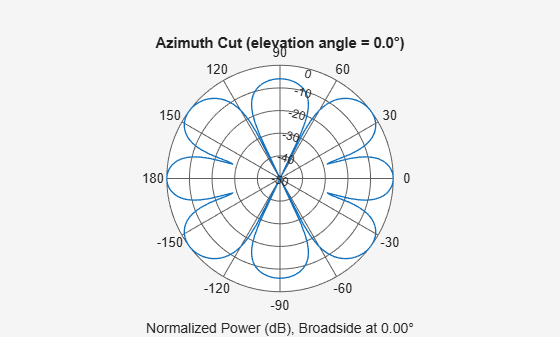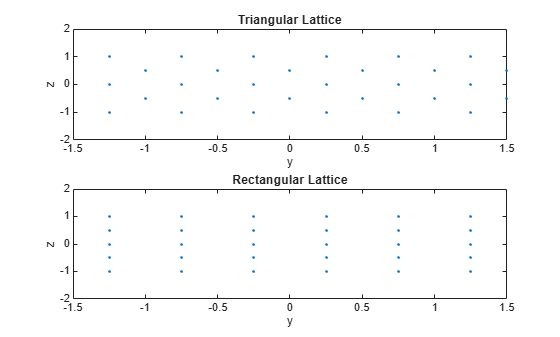# phased.URA

Uniform rectangular array

## Description

The `URA` object constructs a uniform rectangular array (URA).

To compute the response for each element in the array for specified directions:

1. Define and set up your uniform rectangular array. See Construction.

2. Call `step` to compute the response according to the properties of `phased.URA`. The behavior of `step` is specific to each object in the toolbox.

### Note

Starting in R2016b, instead of using the `step` method to perform the operation defined by the System object™, you can call the object with arguments, as if it were a function. For example, ```y = step(obj,x)``` and `y = obj(x)` perform equivalent operations.

## Construction

`H = phased.URA` creates a uniform rectangular array System object, `H`. The object models a URA formed with identical sensor elements. Array elements are distributed in the yz-plane in a rectangular lattice. The array look direction (boresight) is along the positive x-axis.

`H = phased.URA(Name,Value)` creates the object, `H`, with each specified property Name set to the specified Value. You can specify additional name-value pair arguments in any order as (`Name1`,`Value1`,...,`NameN`,`ValueN`).

`H = phased.URA(SZ,D,Name,Value)` creates a URA object, `H`, with the `Size` property set to `SZ`, the `ElementSpacing` property set to `D` and other specified property Names set to the specified Values. `SZ` and `D` are value-only arguments. When specifying a value-only argument, specify all preceding value-only arguments. You can specify name-value pair arguments in any order.

## Properties

`Element`

Phased array toolbox system object

Element specified as a Phased Array System Toolbox object. This object can be an antenna or microphone element.

Default: Isotropic antenna element with default properties

`Size`

Size of array

A 1-by-2 integer vector or a single integer containing the size of the array. If `Size` is a 1-by-2 vector, the vector has the form `[NumberOfRows, NumberOfColumns]`. If `Size` is a scalar, the array has the same number of elements in each row and column. For a URA, array elements are indexed from top to bottom along a column and continuing to the next columns from left to right. In this illustration, a `'Size'` value of `[3,2]` array has three rows and two columns.Default: `[2 2]`

`ElementSpacing`

Element spacing

A 1-by-2 vector or a scalar containing the element spacing of the array, expressed in meters. If `ElementSpacing` is a 1-by-2 vector, it is in the form of `[SpacingBetweenRows,SpacingBetweenColumns]`. See Spacing Between Columns and Spacing Between Rows. If `ElementSpacing` is a scalar, both spacings are the same.

Default: `[0.5 0.5]`

`Lattice`

Element lattice

Specify the element lattice as one of ```'Rectangular' | 'Triangular'```. When you set the `Lattice` property to `'Rectangular'`, all elements in the URA are aligned in both row and column directions. When you set the `Lattice` property to `'Triangular'`, elements in even rows are displaced toward the positive row axis direction. The displacement is one-half the element spacing along the row.

Default: `'Rectangular'`

`ArrayNormal`

Array normal direction

Array normal direction, specified as one of `'x'`, `'y'`, or `'z'`.

URA elements lie in a plane orthogonal to the selected array normal direction. Element boresight directions point along the array normal direction

ArrayNormal Property ValueElement Positions and Boresight Directions
`'x'`Array elements lie on the yz-plane. All element boresight vectors point along the x-axis.
`'y'`Array elements lie on the zx-plane. All element boresight vectors point along the y-axis.
`'z'`Array elements lie on the xy-plane. All element boresight vectors point along the z-axis.

Default: `'x'`

`Taper`

Element tapers

Element tapers, specified as a complex-valued scalar, or 1-by-MN row vector, MN-by-1 column vector, or M-by-N matrix. Tapers are applied to each element in the sensor array. Tapers are often referred to as element weights. M is the number of elements along the z-axis, and N is the number of elements along y-axis. M and N correspond to the values of `[NumberofRows, NumberOfColumns]` in the `Size` property. If `Taper` is a scalar, the same taper value is applied to all elements. If the value of `Taper` is a vector or matrix, taper values are applied to the corresponding elements. Tapers are used to modify both the amplitude and phase of the received data.

Default: 1

## Methods

 collectPlaneWave Simulate received plane waves directivity Directivity of uniform rectangular array getElementNormal Normal vector to array elements getElementPosition Positions of array elements getNumElements Number of elements in array getTaper Array element tapers isPolarizationCapable Polarization capability pattern Plot URA array pattern patternAzimuth Plot URA array directivity or pattern versus azimuth patternElevation Plot URA array directivity or pattern versus elevation plotGratingLobeDiagram Plot grating lobe diagram of array plotResponse Plot response pattern of array step Output responses of array elements viewArray View array geometry
Common to All System Objects
`release`

Allow System object property value changes

## Examples

collapse all

Construct a 3-by-2 rectangular lattice URA. By default, the array consists of isotropic antenna elements. Find the response of each element at boresight, 0 degrees azimuth and elevation. Assume the operating frequency is 1 GHz.

```array = phased.URA('Size',[3 2]); fc = 1e9; ang = [0;0]; resp = array(fc,ang); disp(resp)```
``` 1 1 1 1 1 1 ```

Plot the azimuth pattern of the array.

```c = physconst('LightSpeed'); pattern(array,fc,[-180:180],0,'PropagationSpeed',c, ... 'CoordinateSystem','polar','Type','powerdb','Normalize',true)```This example shows how to find and plot the positions of the elements of a 5-row-by-6-column URA with a triangular lattice and a URA with a rectangular lattice. The element spacing is 0.5 meters for both lattices.

Create the arrays.

```h_tri = phased.URA('Size',[5 6],'Lattice','Triangular'); h_rec = phased.URA('Size',[5 6],'Lattice','Rectangular');```

Get the element y,z positions for each array. All the x coordinates are zero.

```pos_tri = getElementPosition(h_tri); pos_rec = getElementPosition(h_rec); pos_yz_tri = pos_tri(2:3,:); pos_yz_rec = pos_rec(2:3,:);```

Plot the element positions in the yz-plane.

```figure; gcf.Position = [100 100 300 400]; subplot(2,1,1); plot(pos_yz_tri(1,:), pos_yz_tri(2,:), '.') axis([-1.5 1.5 -2 2]) xlabel('y'); ylabel('z') title('Triangular Lattice') subplot(2,1,2); plot(pos_yz_rec(1,:), pos_yz_rec(2,:), '.') axis([-1.5 1.5 -2 2]) xlabel('y'); ylabel('z') title('Rectangular Lattice')```Construct a 5-by-2 element URA with a Taylor window taper along each column. The tapers form a 5-by-2 matrix.

```taper = taylorwin(5); ha = phased.URA([5,2],'Taper',[taper,taper]); w = getTaper(ha)```
```w = 10×1 0.5181 1.2029 1.5581 1.2029 0.5181 0.5181 1.2029 1.5581 1.2029 0.5181 ```

expand all

## References

 Brookner, E., ed. Radar Technology. Lexington, MA: LexBook, 1996.

 Brookner, E., ed. Practical Phased Array Antenna Systems. Boston: Artech House, 1991.

 Mailloux, R. J. “Phased Array Theory and Technology,” Proceedings of the IEEE, Vol., 70, Number 3s, pp. 246–291.

 Mott, H. Antennas for Radar and Communications, A Polarimetric Approach. New York: John Wiley & Sons, 1992.

 Van Trees, H. Optimum Array Processing. New York: Wiley-Interscience, 2002.